# What are 2

What are the two more terms of the GP a, ax, ax2, ax3, __, __?

Result

a5 = (Correct answer is: ax^4)a6 = (Correct answer is: ax^5)### Step-by-step explanation: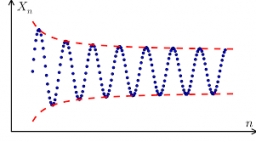Did you find an error or inaccuracy? Feel free to write us. Thank you!## Related math problems and questions:

• Evaluate expr with 2 varsIf a=6 and x=2, find the value of (2ax + 7x- 10) /(4ax-3a-2)
• Sum of GP members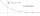Determine the sum of the GP 30, 6, 1.2, to 5 terms. What is the sum of all terms (to infinity)?
• Missing term 2What is the missing term for the Geometric Progression (GP) 3, 15, 75,__, 1875?
• GP membersThe geometric sequence has 10 members. The last two members are 2 and -1. Which member is -1/16?
• GP - three membersThe second and third of a geometric progression are 24 and 12(c+1), respectively, given that the sum of the first three terms of progression is 76. determine the value of c.
• GP - sequence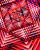The first three terms of a geometric sequence are as follows 10, 30. 90, find the next two terms of this sequence.
• Geometric progressiobIf the sum of four consective terms of geometric progression is 80 and arithmetic mean of second and fourth term is 30 then find terms?
• What is 10What is the 5th term, if the 8th term is 80 and common ratio r =1/2?
• Terms of GPWhat is the 6th term of the GP 9, 81, 729,. .. ?
• Q of GP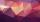Calculate quotient of geometric progression if a1=5 a a1+a2=12.
• Two geometric progressions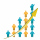Insert several numbers between numbers 6 and 384 so that they form with the given GP numbers and that the following applies: a) the sum of all numbers is 510 And for another GP to apply: b) the sum of entered numbers is -132 (These are two different geome
• Arithmetic progression 2The 3rd term of an Arithmetic progression is ten more than the first term, while the fifth term is 15 more than the second term. Find the sum of the 8th and 15th terms of the Arithmetic progression if the 7th term is seven times the first term.
• Six termsFind the first six terms of the sequence a1 = -3, an = 2 * an-1
• If the 3If the 6th term of a GP is 4 and the 10th is 4/81, find common ratio r.
• Geometric progressionIn geometric progression, a1 = 7, q = 5. Find the condition for n to sum first n members is sn≤217.
• What areWhat are the four terms between 4 and 19?
• GP - 8 itemsDetermine the first eight members of a geometric progression if a9=512, q=2